# python数据清洗系列之字符串处理详解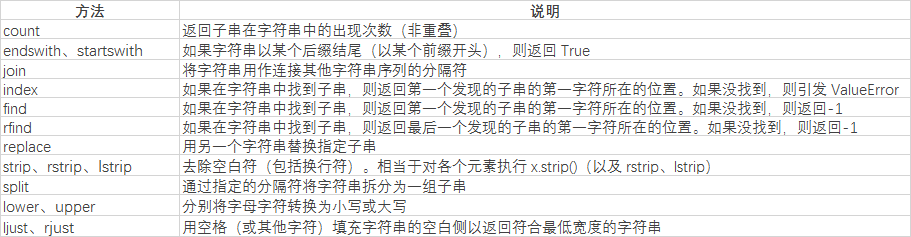```str='i like apple,i like bananer'
print(str.split(','))```

['i like apple', 'i like bananer']

`print(str.split(' '))`

['i', 'like', 'apple,i', 'like', 'bananer']

```print(str.index(','))
print(str.find(','))```

12

`print(str.count('i'))`

4

connt用于统计目标字符串的频率

`print(str.replace(',', ' ').split(' '))`

['i', 'like', 'apple', 'i', 'like', 'bananer']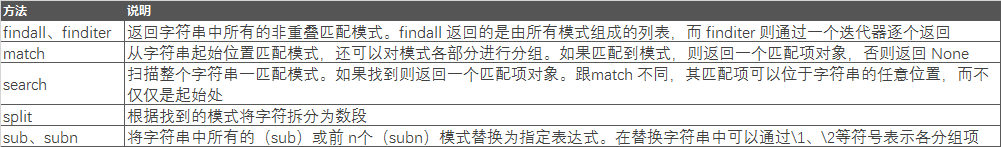```str = "Cats are smarter than dogs"
pattern=re.compile(r'(.*) are (.*?) .*')
result=re.match(pattern,str)

for i in range(len(result.groups())+1):
print(result.group(i))```

Cats are smarter than dogs
Cats
smarter

`pattern=re.compile(r'are (.*?) .*')`

match则返回none，search返回结果为：

are smarter than dogs
smarter

```str = "138-9592-5592 # number"
pattern=re.compile(r'#.*\$')
number=re.sub(pattern,'',str)
print(number)```

138-9592-5592

`print(re.sub(r'-*','',number))`

13895925592

```str = "138-9592-5592 # number"
pattern=re.compile(r'5')
print(pattern.findall(str))```

['5', '5', '5']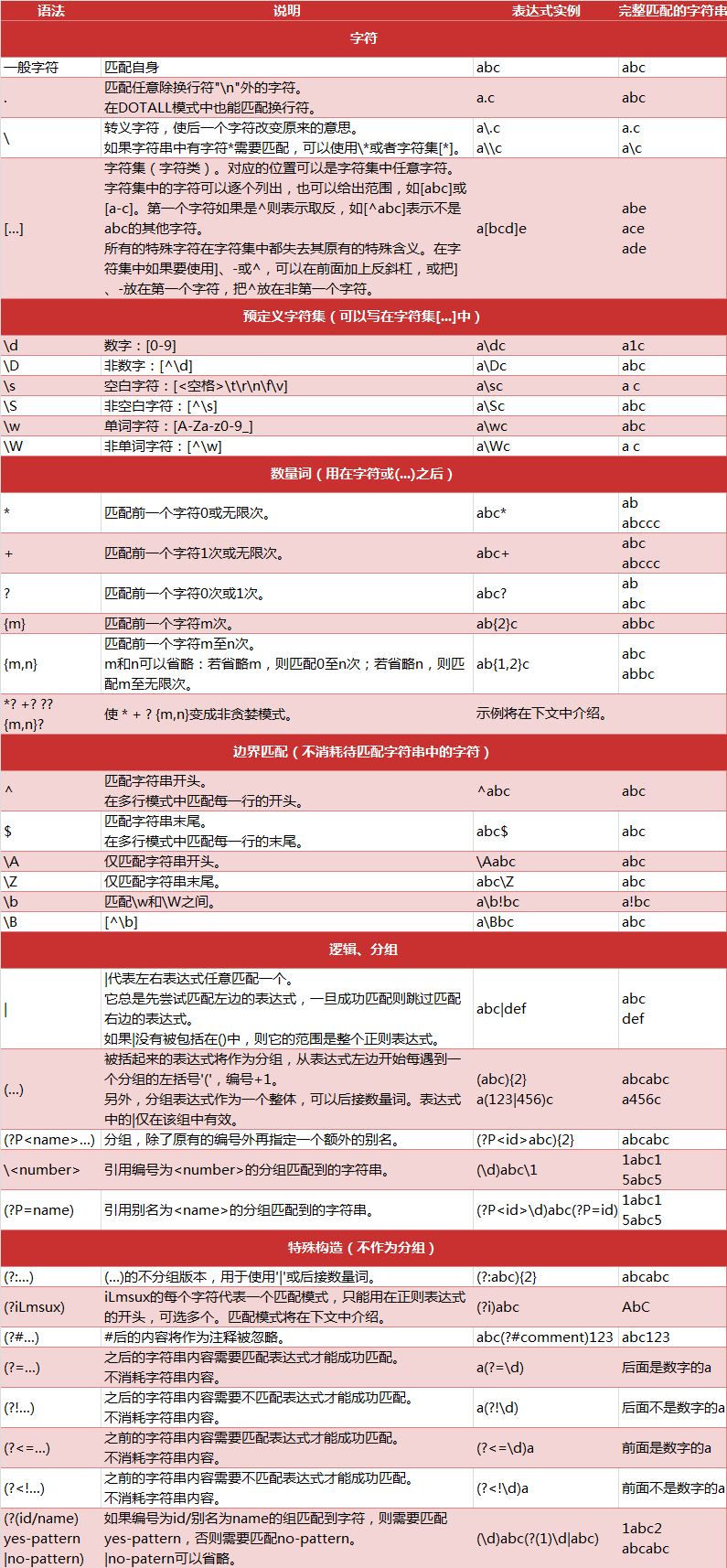```data = pd.Series({'li': '120@qq.com','wang':'5632@qq.com',
'chen': '8622@xinlang.com','zhao':np.nan,'sun':'5243@gmail.com'})
print(data)```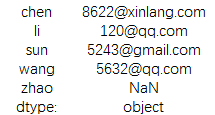`print(data.str.contains('@'))`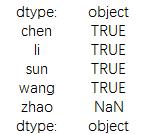```data = pd.Series({'li': '120@qq.com','wang':'5632@qq.com',
'chen': '8622@xinlang.com','zhao':np.nan,'sun':'5243@gmail.com'})
pattern=re.compile(r'(d*)@([a-z]+).([a-z]{2,4})')
result=data.str.match(pattern) #这里用fillall的方法也可以result=data.str.findall(pattern)
print(result)```

chen [(8622, xinlang, com)]
li [(120, qq, com)]
sun [(5243, gmail, com)]
wang [(5632, qq, com)]
zhao NaN
dtype: object

`print(result.str.get(0))`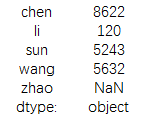`print(result.str.get(1))`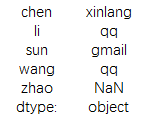```data = pd.Series({'li': '120@qq.com','wang':'5632@qq.com',
'chen': '8622@xinlang.com','zhao':np.nan,'sun':'5243@gmail.com'})
print(data.str[:6])```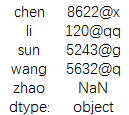python cx_Oracle模块的安装和使用详细介绍
pythoncx_Oracle模块的安装最近需要写一个数据迁移脚本，将单一Oracle中的数据迁移到MySQLSharding集群，在linux下安装cx_Oracle感觉还是有一点麻烦的，整理一

Python IDLE 错误：IDLE''s subprocess didn''t make connection 的解决方案
PythonIDLE错误描述：SubprocessStartupErrorIDLE'ssubprocessdidn'tmakeconnection.EitherIDLEcan'tstartasubprocessorpersonalfirewallsoftwareisblockingtheconnection.错误截图：错误原因分析

Python中类型检查的详细介绍

Copyright © 2010 Gimoo.Net. All Rights Rreserved  京ICP备05050695号